# ICS 312 LEX Set 25 LEX Lex is

• Slides: 27ICS 312 LEX Set 25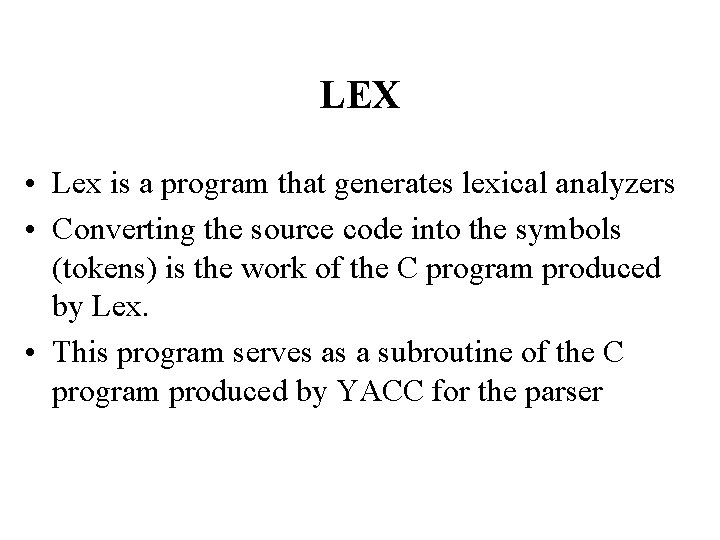LEX • Lex is a program that generates lexical analyzers • Converting the source code into the symbols (tokens) is the work of the C program produced by Lex. • This program serves as a subroutine of the C program produced by YACC for the parser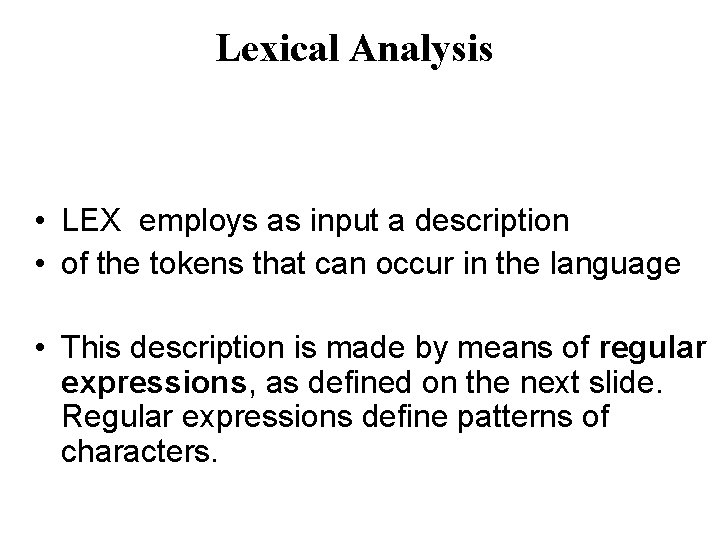Lexical Analysis • LEX employs as input a description • of the tokens that can occur in the language • This description is made by means of regular expressions, as defined on the next slide. Regular expressions define patterns of characters.Basics of Regular Expressions 1. Any character (or string of characters) except those (called metacharacters) which have a special interpretation, such as () [] {} + * ? | etc. For instance the string “if” in a regular expression will match the identical string in the source code.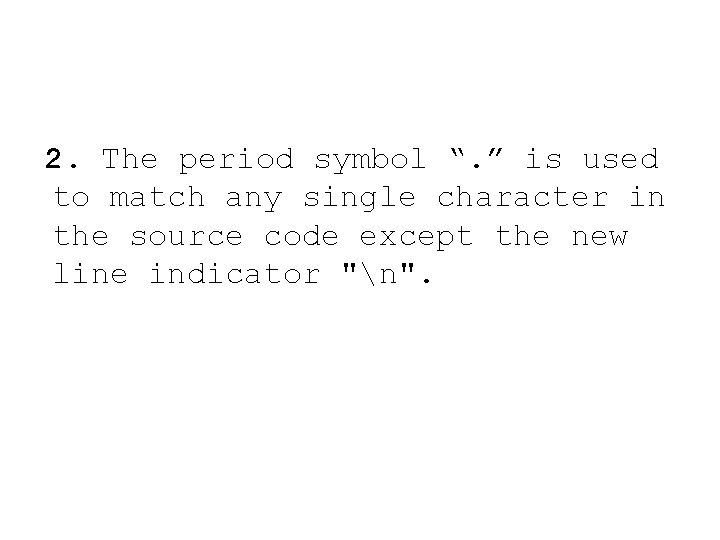2. The period symbol “. ” is used to match any single character in the source code except the new line indicator "n".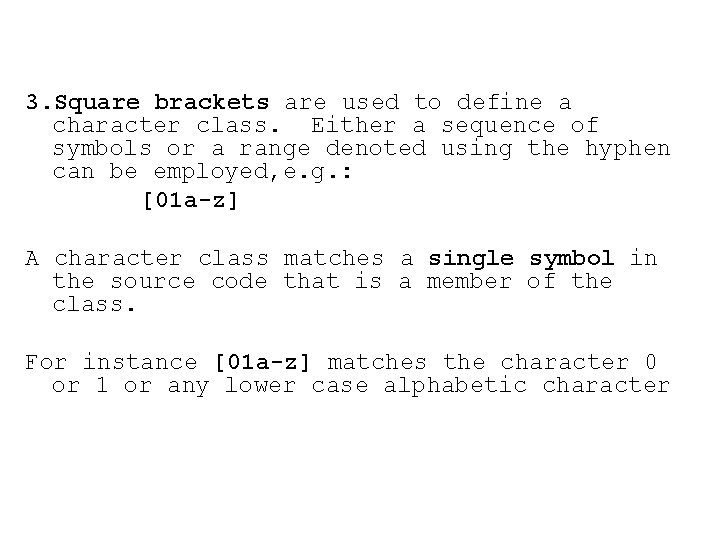3. Square brackets are used to define a character class. Either a sequence of symbols or a range denoted using the hyphen can be employed, e. g. : [01 a-z] A character class matches a single symbol in the source code that is a member of the class. For instance [01 a-z] matches the character 0 or 1 or any lower case alphabetic character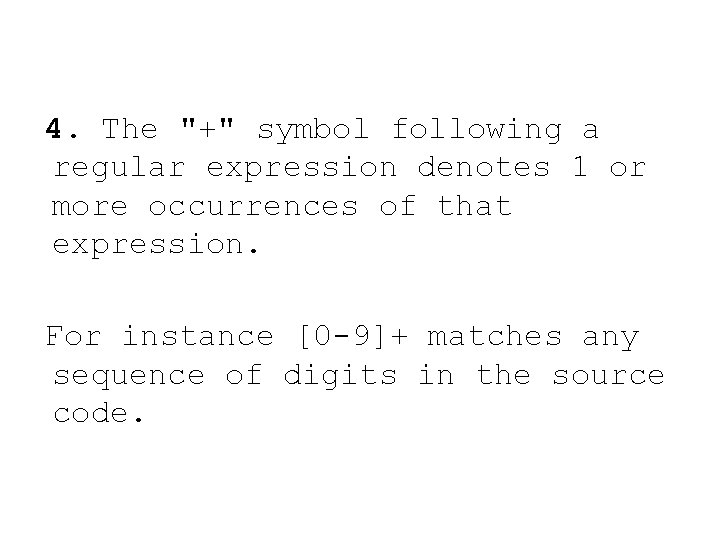4. The "+" symbol following a regular expression denotes 1 or more occurrences of that expression. For instance [0 -9]+ matches any sequence of digits in the source code.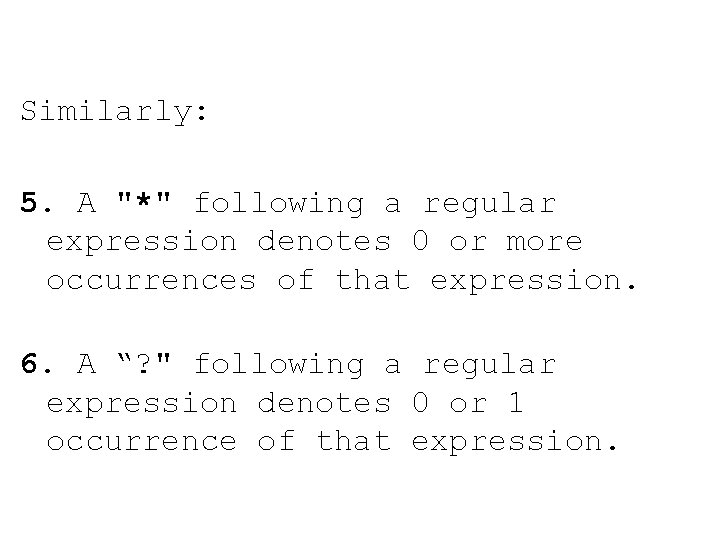Similarly: 5. A "*" following a regular expression denotes 0 or more occurrences of that expression. 6. A “? " following a regular expression denotes 0 or 1 occurrence of that expression.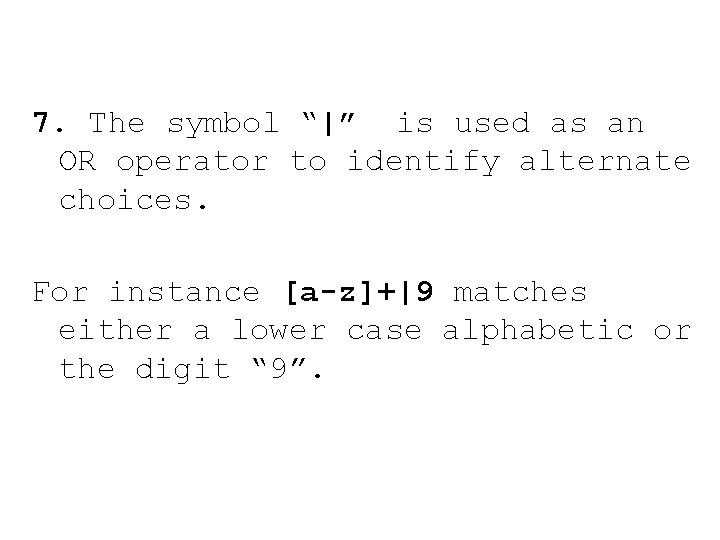7. The symbol “|” is used as an OR operator to identify alternate choices. For instance [a-z]+|9 matches either a lower case alphabetic or the digit “ 9”.8. Parentheses can be freely used. For example: (a|b)+ matches e. g. abba while a|b+ match a or a string of b’s.9. Regular expressions can be concatenated For instance: [a-z. A-Z]*[0 -9]+[a-z. A-Z] matches any sequence of 0 or more letters, followed by 1 or more digits, followed by 1 letter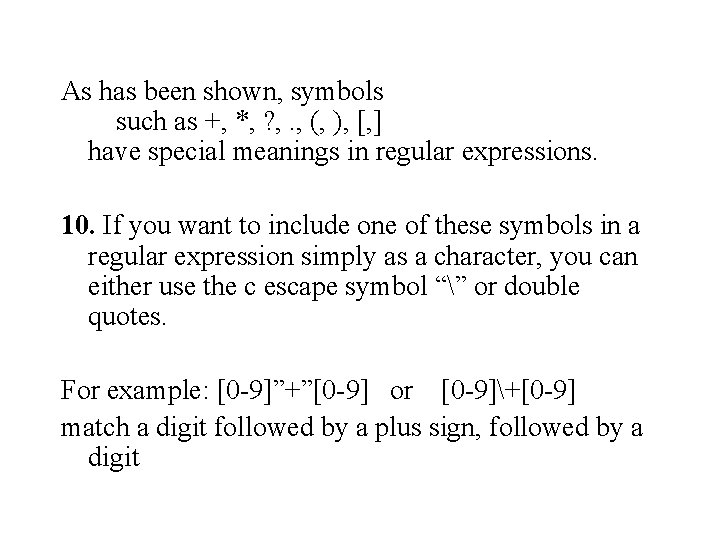As has been shown, symbols such as +, *, ? , . , (, ), [, ] have special meanings in regular expressions. 10. If you want to include one of these symbols in a regular expression simply as a character, you can either use the c escape symbol “” or double quotes. For example: [0 -9]”+”[0 -9] or [0 -9]+[0 -9] match a digit followed by a plus sign, followed by a digitExamples Given: R = ( abb | cd ) and S = abc RS = ( abbabc | cdabc ) is a regular expression. SR = ( abcabb | abccd ) is a regular expression. The following strings are matched by R*: abbcdcd e cdabbabbcd abb cd cdcdcdcd and so forth.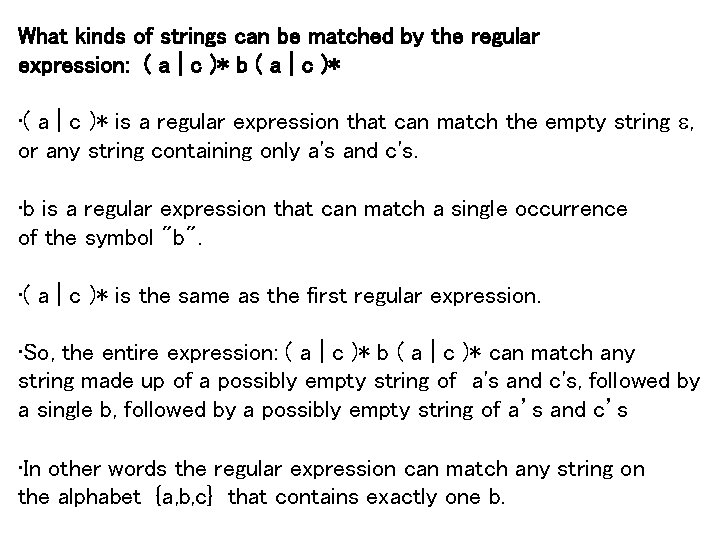What kinds of strings can be matched by the regular expression: ( a | c )* b ( a | c )* • ( a | c )* is a regular expression that can match the empty string e, or any string containing only a's and c's. • b is a regular expression that can match a single occurrence of the symbol "b". • ( a | c )* is the same as the first regular expression. • So, the entire expression: ( a | c )* b ( a | c )* can match any string made up of a possibly empty string of a's and c's, followed by a single b, followed by a possibly empty string of a’s and c’s • In other words the regular expression can match any string on the alphabet {a, b, c} that contains exactly one b.What kinds of strings can be matched by the regular expression: ( a | c )* ( b | e ) ( a | c )* • This is the same as the previous example, except that the regular expression in the center is now: ( b | e ) • ( b | e ) can match either an occurrence of a single b, or the empty string which contains no characters • So the entire expression ( a | c )* ( b | e ) ( a | c )* can match any string over the alphabet {a, b, c} that contains either 0 or 1 b's.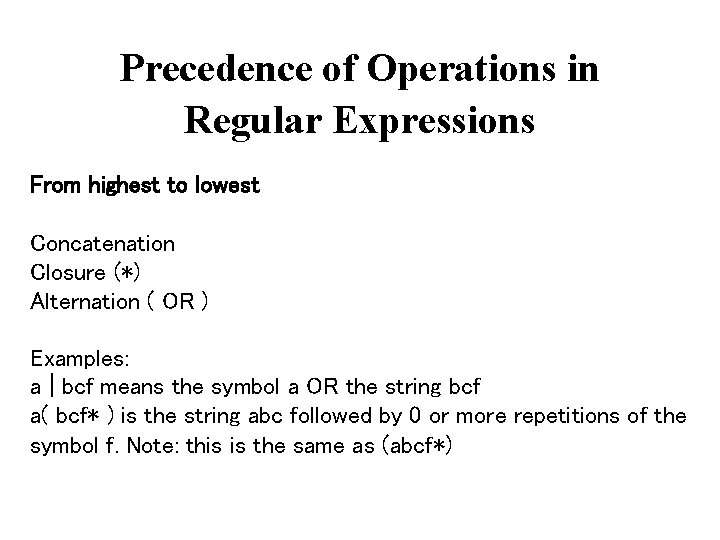Precedence of Operations in Regular Expressions From highest to lowest Concatenation Closure (*) Alternation ( OR ) Examples: a | bcf means the symbol a OR the string bcf a( bcf* ) is the string abc followed by 0 or more repetitions of the symbol f. Note: this is the same as (abcf*)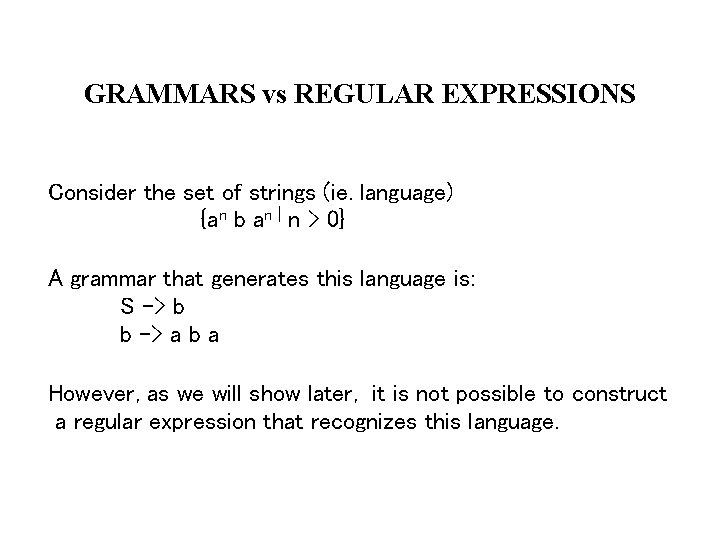GRAMMARS vs REGULAR EXPRESSIONS Consider the set of strings (ie. language) {an b an | n > 0} A grammar that generates this language is: S -> b b -> a b a However, as we will show later, it is not possible to construct a regular expression that recognizes this language.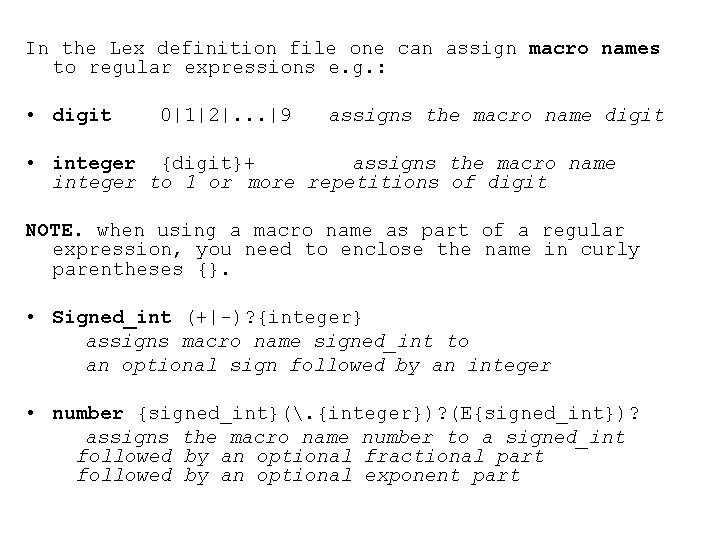In the Lex definition file one can assign macro names to regular expressions e. g. : • digit 0|1|2|. . . |9 assigns the macro name digit • integer {digit}+ assigns the macro name integer to 1 or more repetitions of digit NOTE. when using a macro name as part of a regular expression, you need to enclose the name in curly parentheses {}. • Signed_int (+|-)? {integer} assigns macro name signed_int to an optional sign followed by an integer • number {signed_int}(. {integer})? (E{signed_int})? assigns the macro name number to a signed_int followed by an optional fractional part followed by an optional exponent part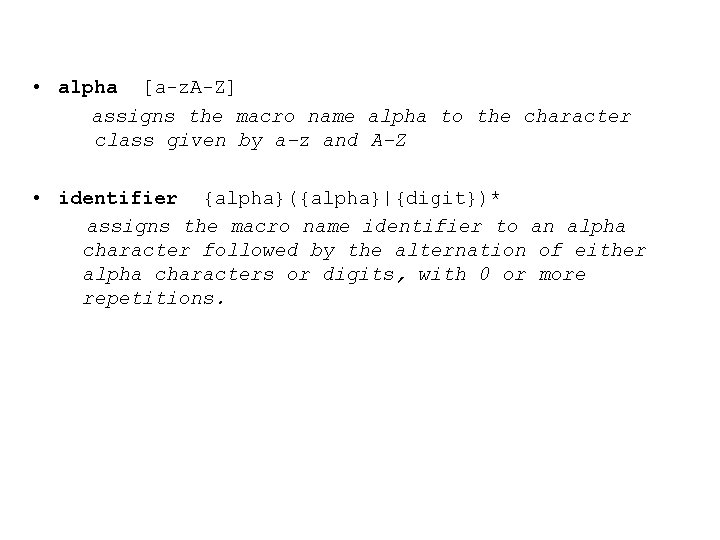• alpha [a-z. A-Z] assigns the macro name alpha to the character class given by a-z and A-Z • identifier {alpha}({alpha}|{digit})* assigns the macro name identifier to an alpha character followed by the alternation of either alpha characters or digits, with 0 or more repetitions.RULE Using the regular expression for an identifier on the previous slide, what would be the first token of the following string? MAX 23= Z 29 + 8 Lex picks as the "next" token, the longest string that can be matched by one of it regular expressions. In this case, MAX 23 would be matched as an identifier, not just M or MAX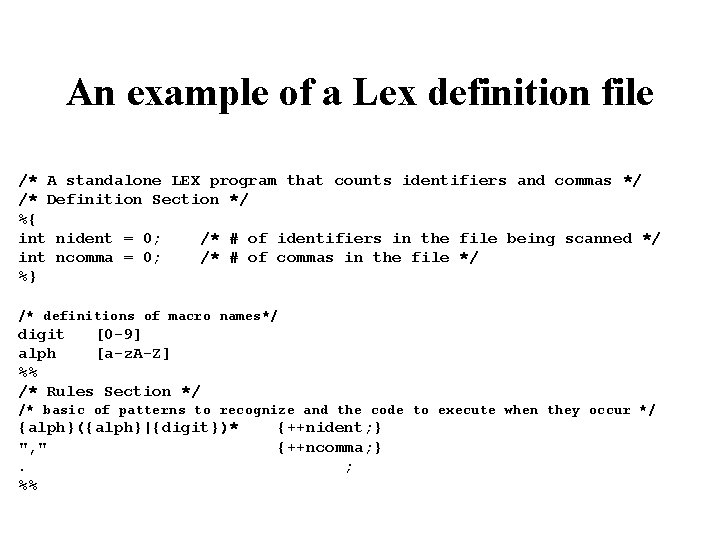An example of a Lex definition file /* A standalone LEX program that counts identifiers and commas */ /* Definition Section */ %{ int nident = 0; /* # of identifiers in the file being scanned */ int ncomma = 0; /* # of commas in the file */ %} /* definitions of macro names*/ digit [0 -9] alph [a-z. A-Z] %% /* Rules Section */ /* basic of patterns to recognize and the code to execute when they occur */ {alph}({alph}|{digit})* {++nident; } ", " {++ncomma; } . ; %%An example of a scanner definition file (Cont. ) /* subroutine section */ /* the last part of the file contains user defined code, as shown here. */ main() { yylex(); printf( "%s%dn", "The no. of identifiers = ", nident); printf( "%s%dn", "The no. of commas = ", ncomma); } /* LEX calls this function when the end of the input file is reached */ yywrap(){}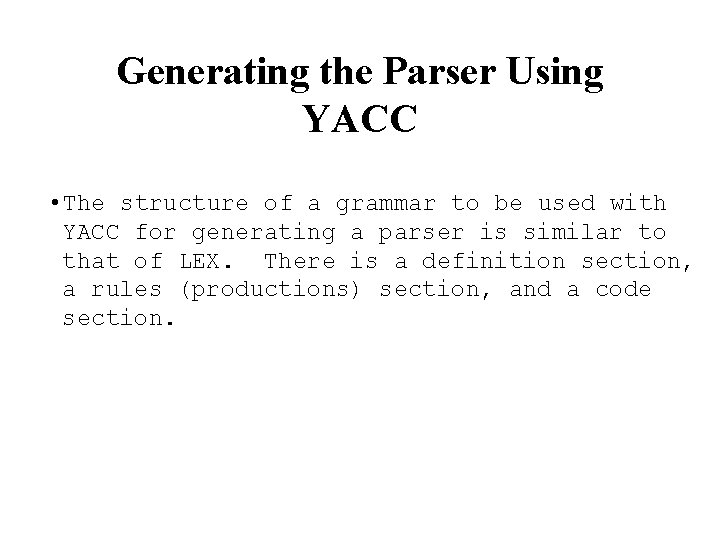Generating the Parser Using YACC • The structure of a grammar to be used with YACC for generating a parser is similar to that of LEX. There is a definition section, a rules (productions) section, and a code section.Example of an Input Grammar for YACC %{ /* ARITH. Y Yacc input for a arithmetic expression evaluator */ #include <stdio. h> /* for printf */ #define YYSTYPE int yyparse(void); int yylex(void); void yyerror(char *mes); %} %token number %%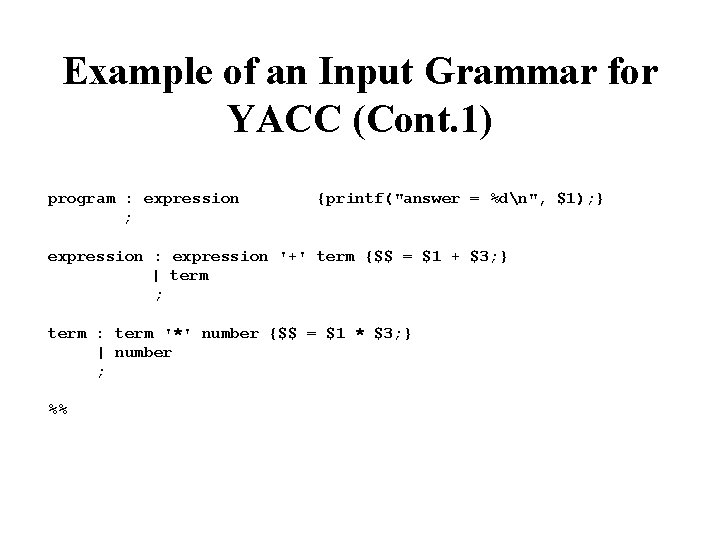Example of an Input Grammar for YACC (Cont. 1) program : expression {printf("answer = %dn", \$1); } ; expression : expression '+' term {\$\$ = \$1 + \$3; } | term ; term : term '*' number {\$\$ = \$1 * \$3; } | number ; %%Example of an Input Grammar for YACC (Cont. 2) void main() { printf("Enter an arithmetic expressionn"); yyparse(); } /* prints an error message */ void yyerror(char *mes) {printf("%sn", mes); }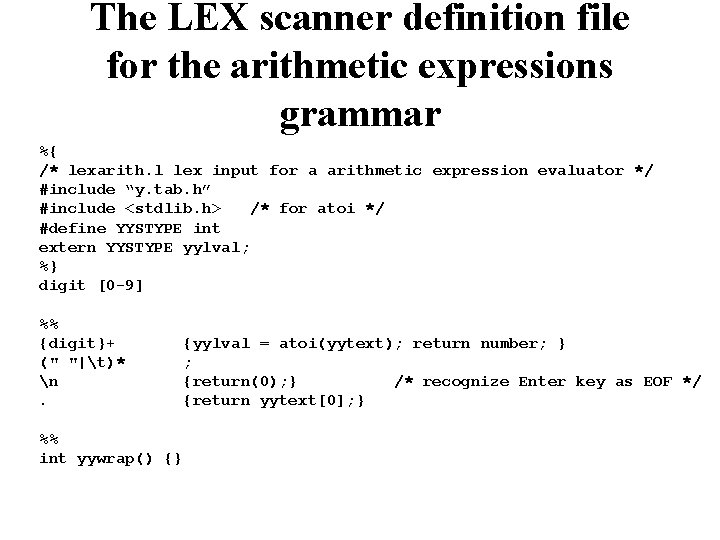The LEX scanner definition file for the arithmetic expressions grammar %{ /* lexarith. l lex input for a arithmetic expression evaluator */ #include “y. tab. h” #include <stdlib. h> /* for atoi */ #define YYSTYPE int extern YYSTYPE yylval; %} digit [0 -9] %% {digit}+ {yylval = atoi(yytext); return number; } (" "|t)* ; n {return(0); } /* recognize Enter key as EOF */ . {return yytext; } %% int yywrap() {}